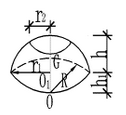球带体积与表面积在线计算器

App说明$$V= \frac {πh}{b} (3r1^2+3r2^2+h^2)$$

$$S1=2πRh$$

$$S=2πRh+π(r1^2+r2^2)$$

• 式中
• R——球半径
• r1，r2——地面半径
• h——腰高
• h1——求心O至带底圆心O1的距离

使用示例

h—腰高：8

R—球半径：4

r1—底面半径：3

r2—底面半径：6

h1—球心O至带底圆心O1的距离：9

Comment list ( 0 )